# How do you control a 500A contactor with a Raspberry Pi?

In this article, we'll cover the basics of controlling contactors with your raspberry pi. Contactors are used to switch large voltages/currents, here we're using a 900V / 500A contactor! You can check out its specifications here:

e.g. KILOVAC LEV200 1 Pole Contactor

Note - I'm using this as an example as I had one lying around, the information also applies if you are controlling relays or any other coil operated devices including hydraulic coils.

There are 4 connections for this, two for controlling the load (large studs) and 2 for powering the coil to bring in the relay. Looking at the coil specification part of the datasheet we can see we need to supply at least 19V to pull in the contactor initially, at this voltage we'll also need to supply at least 0.47A as well to bring the contactor in.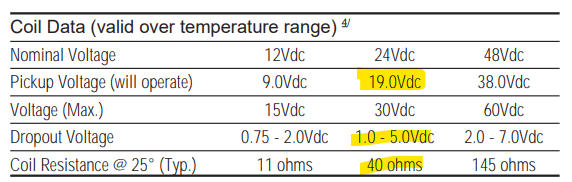The PI GPIO can control up to about 16mA at 3v3 so we'll obviously need some additional circuitry to do this.

We'll use a HAT to do this since the raspberry PI GPIO can't control high voltage / current loads, we'll also want some terminal blocks as well as a method for mounting everything securely which most HATs provide. In this example, I've used the following HAT https://piio.co.uk/piio-do/

This HAT gives us 24 channels that can switch up to 24V at 0.5A, in addition since it uses MOSFETS to do this we can also PWM the outputs which we'll use to save power once the contactor is pulled in. Looking at the coil data we can see there is something called 'dropout voltage' this means that once the contactor has been pulled in we only need to supply a much lower voltage to keep the contactor pulled in. Since the voltage is approx 5x less than the pull-in voltage the power loss would also be much less.

• P (pullin) = (24 x 24) / 40 = 14.4W
• P (hold) = (5 x 5) / 40 = 0.625W

Notice that power loss in the relay is proportional to the square of the voltage divided by the coil resistance! So Being able to reduce the voltage reduces power lost in an exponential manner. Here a 4x reduction in voltage gives us a 23x reduction in power losses! In some applications, you aren't going to care about this too much but if you are operating off a battery or are operating in an enclosure that would be heated up by all this it can become quite critical.

There's an illustration here of how we intend to apply a fixed then PWM'd voltage to control the average voltage and current to the coil after a fixed delay to let the contactor pull in (time periods changed for clarity).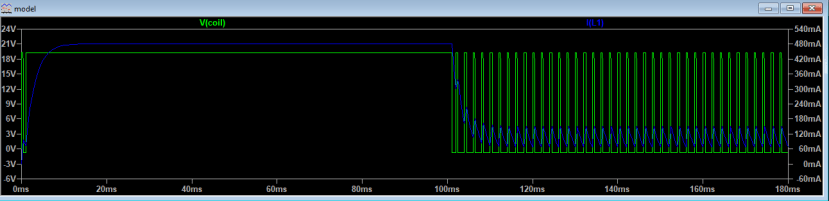Combining the two gives us the power losses in the coil, and how much we can save by this scheme.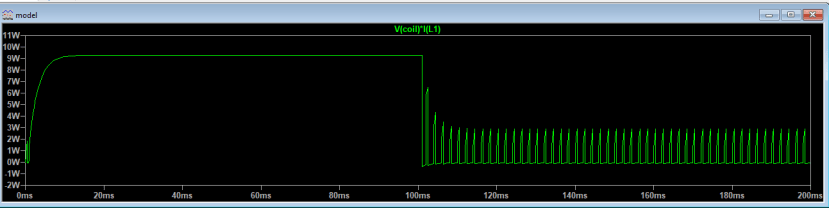So by controlling the voltage to the contactor intelligently we have the opportunity to save a lot of power. What we do now is wire up the contactor to our HAT, we'll use python to control the contactor and power everything using a 20V supply (pi uses a separate 5V supply here). The code will bring in the contactor at full voltage then shortly after switch to a low power mode of operation to reduce power loss in the contactor actuation solenoid.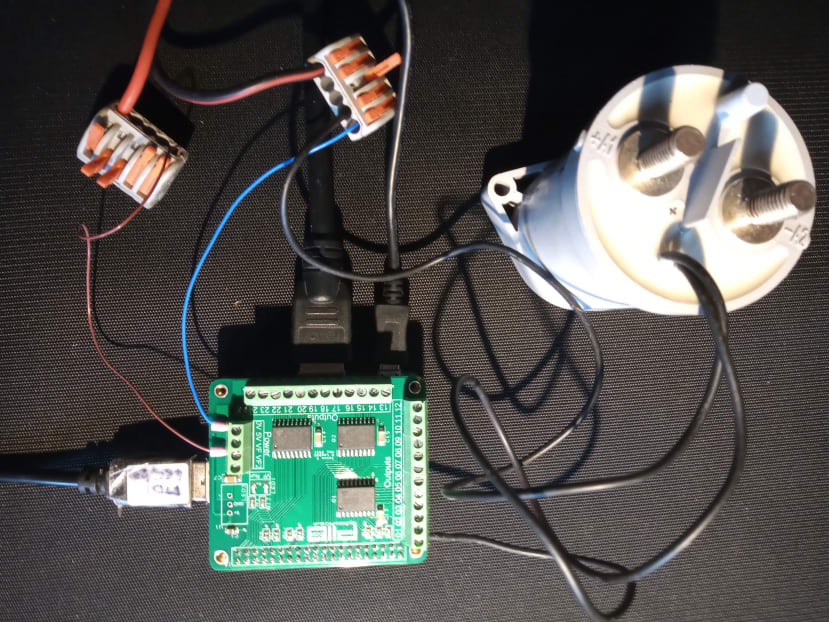We'll use python to demo this as well as the GPIO zero library which allows us to control GPIO as PWM on the PI. We'll set the duty cycle to 100% then 25% which will give us a voltage to the coil of 20 ... then 5V.

We'll need to declare the output as a PWM type to be able to do this.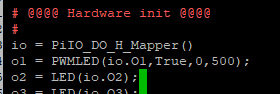Then we just need to generate a delay to set the output to values that pull in, then hold the contactor in.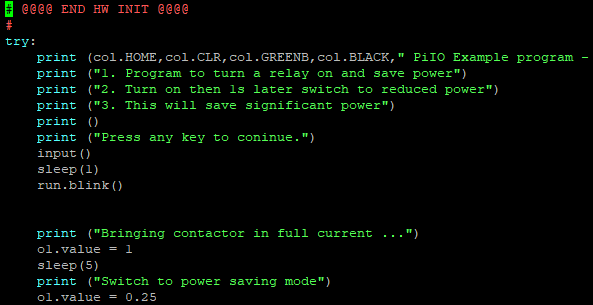GPIO Zero allows us to do this with the '.value' field, we've chosen 65 to be sure the coil remains pulled in to be sure the solution is robust. In the coil specification, the coil resistance is specified at 25 degrees, as we pass current through it its resistance increases so we'd like a bit of headroom to take into account supply fluctuations as well as variations in temperature.

We can then run the program and see the impressive power savings! We also have the opportunity of lowering the power losses much further by experimenting to see the real dropout voltage of the contactor by experimentation since the 5V we chose was very conservative.

Also note if we'd decided to use a 24V power supply the initial coil current would have gone up to 0.6A which is greater than the 0.5A the MOSFETs were rated at, to overcome this we could have picked a coil with a higher on resistance or paralleled up the MOSFETS to give a higher switching capacity - I'll cover the options here in Part 2.

Code is available here:

https://github.com/lawsonkeith/

keithl has not written a bio yet…